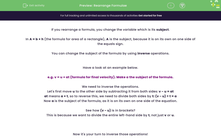# Rearrange Formulae

In this worksheet, students will rearrange formulae (in which subject appears once only) to make different terms the subject using inverse operations.Key stage:  KS 4

Year:  GCSE

GCSE Subjects:   Maths

GCSE Boards:   Pearson Edexcel, OCR, Eduqas, AQA,

Curriculum topic:   Algebra

Curriculum subtopic:   Notation, Vocabulary and Manipulation Algebraic Formulae

Difficulty level:#### Worksheet Overview

If you rearrange a formula, you change the variable which is its subject.

In A = b × h (the formula for area of a rectangle), A is the subject, because it is on its own on one side of the equals sign.

You can change the subject of the formula by using inverse operations.

Have a look at an example below.

e.g. v = u + at (formula for final velocity). Make a the subject of the formula.

We need to inverse the operations.

Let's first move u to the other side by subtracting it from both sides: v - u = at

at means a × t, so to reverse this, we need to divide both sides by t: (v - u) ÷ t = a

Now a is the subject of the formula, as it is on its own on one side of the equation.

See how (v - u) is in brackets?

This is because we want to divide the entire left-hand side by t, not just v or u.

Now it's your turn to inverse those operations!

In this activity, we are going to rearrange formulae to make different terms the subject using inverse operations.

### What is EdPlace?

We're your National Curriculum aligned online education content provider helping each child succeed in English, maths and science from year 1 to GCSE. With an EdPlace account you’ll be able to track and measure progress, helping each child achieve their best. We build confidence and attainment by personalising each child’s learning at a level that suits them.

Get started